## Linear Equations: Solutions Using Determinants with Two Variables

A square array of numbers or variables enclosed between vertical lines is called a determinant. A determinant is different from a matrix in that a determinant has a numerical value, whereas a matrix does not. The following determinant has two rows and two columns.The value of this determinant is found by finding the difference between the diagonally down product and the diagonally up product:##### Example 1

Evaluate the following determinant.##### Example 2

Solve the following system by using determinants.To solve this system, three determinants are created. One is called the denominator determinant, labeled D; another is the x‐numerator determinant , labeled D x; and the third is the y‐numerator determinant , labeled D y .

The denominator determinant, D, is formed by taking the coefficients of x and y from the equations written in standard form.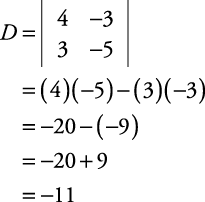The x‐numerator determinant is formed by taking the constant terms from the system and placing them in the x‐coefficient positions and retaining the y‐coefficients.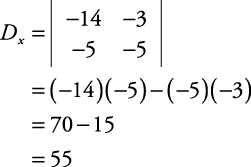The y‐numerator determinant is formed by taking the constant terms from the system and placing them in the y‐coefficient positions and retaining the x‐coefficients.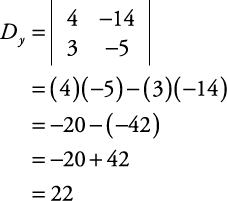The answers for x and y are as follows: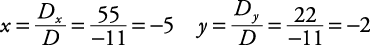The check is left to you. The solution is x = –5, y = –2.

Many times, finding solutions by using determinants is referred to as Cramer's Rule, named after the mathematician who devised this method. Cramer's Rule could hardly be considered a “shortcut,” but it is a rather neat way to solve systems of equations by using determinants.

##### Example 3

Use Cramer's Rule to solve this system.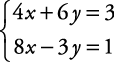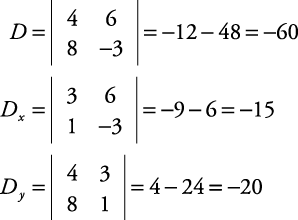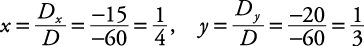The check is left to you. The solution is,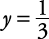.